# Lecture notes

## Transcription

Lecture notes
```Microeconomics I - Lecture #9, April 14, 2009
9
9.1
D/S of/for Labor
Demand for Labor
Demand for labor depends on the price of labor, price of output and production function. In
optimum a firm employs so many units of labor (number of workers) so that the value of marginal
product of labor equals the wage. Look at the following example:
L
1
2
3
4
5
TP MP
30
20
50
16
66
10
76
4
84
P (MR)
4000
VMP
w (MC)
80000
60000
64000
60000
40000
60000
16000
60000
4000
4000
4000
4000
From the data in the table it follows that this firm should hire three employees. It is so because
hiring third employee will increase the profit by 64 000 and the wage of the worker is lower - 60
000. If the firm hires one more worker the fourth worker would bring profit of 40 000 but his wage
would have to be higher - 60 000. So profit maximizing firm would hire three workers. If wage
decreases below 40 000 it would be optimal to hire four workers. If the wage increases above 64
000 it would be better to hire only two workers.
In other words, the firm chooses to hire so many workers that the value of marginal product of
labor equals wage:
V M P L = M PL × P = w
This situation is illustrated on the picture below. If the wage is w0 that the optimal choice for a
firm is to employ L0 workers. The revenue is a shaded rectangle on the left picture below.
43
If the wage increases the optimal level of labor decreases as the worker are more expensive to hire.
The demand for labor is actually identical with V M PL curve. But only up to the point where
wage becomes to high. If the wage is too high firm will stop hiring any workers because the wage
is higher than average revenue from a unit of labor which means that the firm is losing money. In
this case it’s better to stop production. Look at the right picture above. This is the case if wage
is higher than maximal level of V APL , i.e. the point where V M PL crosses V APL .
Up to now we analyzed short-run demand for labor. In short-run all factors of production apart
from labor are fixed. Now we will analyze the demand for labor in long-run. In long-run all factors
of production can be changed. The intuition is the same - if wage increases a firm will hire less
workers. The difference between short- and long-run is the motives for this change. In short run
decreasing demand for labor is caused be decreasing revenue from marginal product of labor. In
long-run decreasing demand for labor is caused by substitution and production effect (similar to
substitution and income effect in consumer optimization problem). This situation is depicted on
the picture below.
9.2
Supply of Labor
Individual supply of labor is the decision between leisure and consumption:
We discussed the choice between two goods. Similarly, we can illustrate the choice between leisure
and consumption. Leisure can be considered as a normal good. Loosely speaking the price of
leisure is forgone earnings, i.e. money that consumer could earn if he spent time working instead
of enjoying leisure time. The optimal choice of consumption and leisure is a combination where the
indifference curve touches the budget line. This choice depends on the wage. If wage increases we
can observe substitution effect (leisure becomes more expensive relative to consumption and should
be substituted by working time and hence higher consumption) and income effect (we assume that
both ”consumption” and ”leisure” are normal goods ⇒ higher income leads to higher consumption
of both goods and hence more leisure). The new optimal choice depends on which of the two effects
is stronger:
44
Market equilibrium minimum wage and taxes: The equilibrium wage on the market is
determined by the point where the supply and demand for labor intercept. Changes in equilibrium
can be caused by changes in demand or supply. If the demand for production increases the demand
for labor increases as well and as a result the equilibrium wage increases as well. Look at the left
hand side of the picture.
If the wages in neighbor countries increase significantly and it is simple to work abroad or if the
wages in similar industries increase the supply of labor curve moves upward and as a result the
equilibrium wage increases. see the right hand side picture above.
Now we will illustrate the effect of income tax on equilibrium wage and number of workers hired.
In the Czech Republic the income tax is 15 - 32%. The situation is depicted on the picture below.
With no taxes the equilibrium employment rate is LE . After the income tax is introduced the
equilibrium employment decreases to LA .
45
Now we analyze the effect of unions. Imagine that unions negotiate the wage increase in a
particular industry from w0 to w1 . Firms are forced to pay more to their workers and as a result
the equilibrium employment decreases (left picture below). Those workers who no longer have a
job in a given industry will go to other industries which means that the supply curve shifts to the
right and as a result the equilibrium wage in the second industry goes down (right picture).
The minimum wage also has an impact on the level of employment. If the minimum wage is
introduced and it is higher than the equilibrium wage than as a result supply of labor is higher
than demand and the unemployment is present.
9.3
D/S of/for Capital
Similarly to decision about the demand for labor a firm will use the level of capital such that the
revenue from marginal product of capital equals its price.
V M PK = v
where v = R + D, R is the forgone interest and D is depreciation or
v = rP + dP
where P is the price of the capital (machine), r is interest rate, and d is depreciation rate.for labor.
As the interest rate increases the demand function for capital decreases and supply increases. The
equilibrium interest rate is given by their intercept.
46
The picture above illustrates the situation when the investment environment improves, i.e. there
are better investment opportunities. This means that firms want to rent more capital and as a
result the the demand curve shifts upwards. The new equilibrium interest rate is higher.
Nominal vs Real interest rate: we have to distinguish between nominal interest rate which
measures change in monetary value of capital and real interest rate which measure change in real
value of capital.
In other words, the nominal interest rate is the amount, in money terms, of interest payable. For
example, suppose a household deposits \$100 with a bank for 1 year and they receive interest of
\$10. At the end of the year their balance is \$110. In this case, the nominal interest rate is 10%
per annum.
The real interest rate, which measures the purchasing power of interest receipts, is calculated by
adjusting the nominal rate charged to take inflation into account. If inflation in the economy has
been 10% in the year, then the \$110 in the account at the end of the year buys the same amount
as the \$100 did a year ago. The real interest rate, in this case, is zero.
The relationship between nominal and real interest rate is:
rN = rR + ie
where rN is nominal interest rate, rR is real interest rate, and ie is expected inflation.
Choice of Investment Projects: there are usually several investment possibilities available.
How do we decide where to invest free capital? We choose the project with the highest value. The
value of project is the present value of all future returns where future returns are discounted to
the present date.
Net present value, NPV, is an indicator of how much value an investment or project adds to
the value of the firm. In financial theory, if there is a choice between two mutually exclusive
alternatives, the one yielding the higher NPV should be selected.
Example: We consider two investment projects. Amount of money necessary to be invested and
returns are summarized below and the interest rate is 10%:
1st year 2nd year 3rd year
A -100000
80000
50000
B -110000
60000
90000
47
80000
50000
= 14050
+
1 + 0.1 (1 + 0.1)2
60000
90000
N P VB = −110000 +
+
= 18925
1 + 0.1 (1 + 0.1)2
N P VA = −100000 +
Since the net present value of project B is higher we should choose project B.
Return vs Risk Investment opportunities bring different returns at different level of risk. More
risky opportunities yield higher return.
Example: An individual can invest into stocks or bonds. Stocks are supposed to bring 20% return
but the investment is risky and this happens only with 50% probability. On the other hand
investment into bonds is safe and yields 8% return with 95% probability. The expected return is
as follows:
ERB = 0.08 × 0.95 = 0.076, i.e. 7.6%
ERS = 0.2 × 0.5 = 0.1, i.e. 10%
We see that expected return of investment into stocks is higher. However, this investment is risky
and some investors would prefer bonds to stocks.
Intertemporal choice
(choice between current and future consumption):
So far we only studied static choices, but life is full of intertemporal choices (should I study for my
test today or tomorrow; should I save or should I consume now, school, cigarettes, alcohol). When
modeling intertemporal choice, economists treat one physical good consumed at two different times
as two different goods.
We consider in this lecture the optimal allocation decision through time. In particular, we examine
the optimal allocation of income to consumption through time. This is important as people often
receive the income through time in a way that does not correspond to their preferred consumption
stream through time. The individual needs to rearrange his or her income stream. This is achieved
by borrowing and saving - through the use of capital markets. We assume here perfect capital
markets, by which is meant that the individual can borrow and save as much as he or she wants
at a constant and given rate of interest, which we shall denote by r . If the rate of interest is 10%
then r = .1; if the rate of interest is 20% then r = .2; and so on. To keep our analysis simple we
assume a two period world. Each individual gets current income Ia and expected future income
Ib and we denote current consumption Ca and future consumption Cb . (For simplicity we assume
a single consumption good and assume a price of 1 in both periods.) It may be the case that the
individual is happy to currently consume his or her income Ia and to consume his or her income Ib
in future. However, the individual may prefer to rearrange his or her consumption by borrowing
or lending. If r is zero the possibilities are obvious: the maximum he or she could consume now
48
is Ia + Ib with zero consumption in future and the maximum he or she could consume in future is
the same. More generally the choice of Ca and Cb must satisfy the budget constraint:
Ca + Cb = Ia + Ib
If there is a positive rate of interest things are a little more complicated. If the individual wanted
to consume nothing now then he or she could save the income Ia , investing it at the rate of interest
r, earning interest rIa and thus in future having
Ia (1 + r) + Ib
Alternatively if he or she wanted to consume nothing in future, then now he or she could spend
his current income plus what he or she could borrow on the strength of being able to pay back in
future.
Ia + Ib /(1 + r)
Generally, the future value of the consumption stream must equal the future value of the income
stream:
Ca (1 + r) + Cb = Ia (1 + r) + Ib
Alternatively, the present value of the consumption stream must equal the present value of the
income stream:
Ca + Cb /(1 + r) = Ia + Ib /(1 + r)
Change of interest rate r: changes the slope of budget constraint. Substitution effect (current
consumption is more expensive) ⇒ higher savings. Income effect (increase in future income) ⇒
higher current consumption. The total effect depends on the shape of indifference curves, i.e. on
whether substitution effect is stronger than income effect or vice versa.
49
```

### \$7.25 EMPLOYEE RIGHTS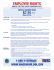More information

### employment application personal employment desired/availability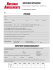More information

### Employment Application - Birch Haven Home Page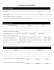More information

### Eastern England, from 1401 to 1700, in quinquennial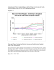More information

### Corey Smith-Employment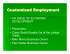More information

### Minimum Wage Slides - Show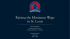More information

### to access original slides from this presentation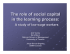More information

### Boletin Legislativo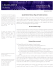More information

### Worker Justice Now, Fall 2015 - Cincinnati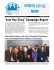More information

### NWPC News Bulletin June2015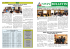More information

### TLR 12 10 14 - The Liscio Report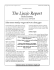More information

### State of the system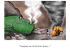More information

### Contact Centre Industry Demographic Survey 2013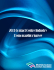More information

### 2nd Edition November2013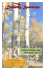More information

### Everybody is entitled to minimum wage - docs.szw.nl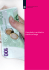More information

### CJ Pony Parts, Inc. - Nevada Governor`s Office of Economic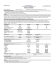More information

### Company answer to CCC survey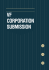More information

### January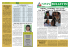More information

### Stallion Inc. - Good Jobs New York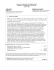More information

### fee ScHeduLe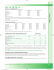More information

### Wage SurveyQuest_2015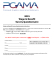More information

### Section 5 - laschools.orgMore information